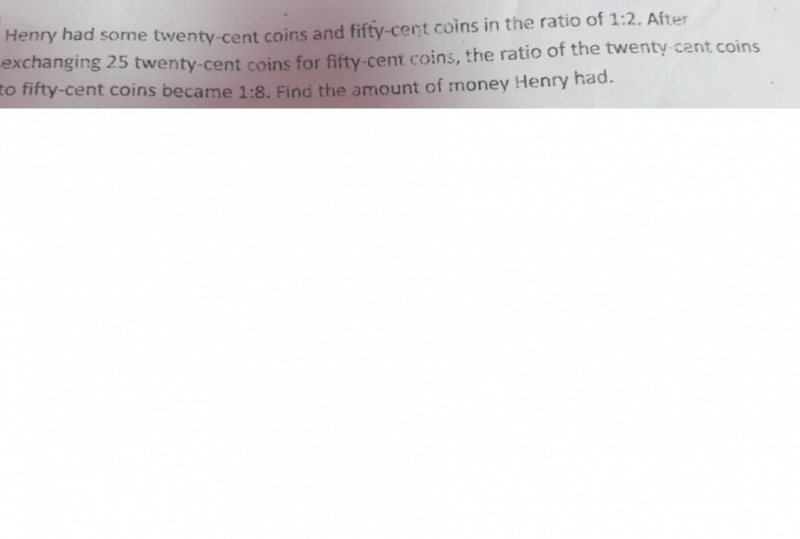# Questioncan you help to solve this problem please?

1 Answer

# Answer

25 × \$0.20 =  \$5
\$5 ÷ \$0.50 = 10

This means that 25 20¢ coins were exchanged for 10 50¢ coins.

 20¢ coins 50¢ coins Before 1 unit 2 units Change -25 +10 After 1 part 8 parts

Making the 20¢ column into 8 parts:

 20¢ coins 50¢ coins Before 8 units 2 units Change -200 +10 After 8 parts 8 parts

8 units – 200 = 2 units +10
8 units – 2 units = 10 + 200
6 units = 210
1 unit = 210 ÷ 6
= 35 (no. of 20¢ coins)
2 units = 35 × 2
= 70 (no. of 50
¢ coins)

35 × \$0.20 = \$7
70 × \$0.50 = \$35
\$35 + \$7 = \$42

0 Replies 0 Likes